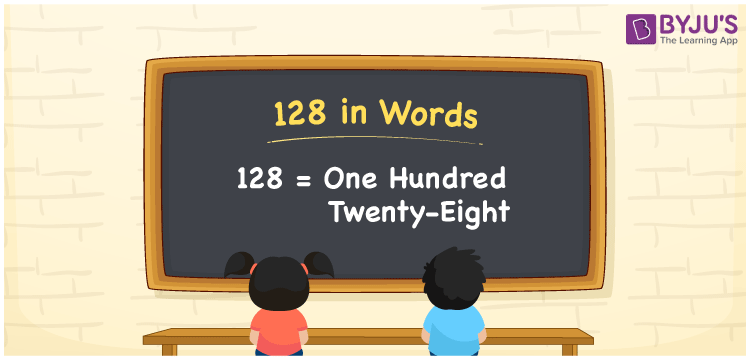# 128 in Words

We can write the number 128 in words as One hundred twenty-eight. In other words, the number name of 128 is One hundred and twenty-eight. Suppose you paid Rs. 128 for a coffee, then you can say, “I paid One hundred and twenty-eight rupees for a coffee”. Also, note that 128 is a cardinal number since it denotes a specific amount or quantity.

 128 in words One hundred twenty-eight One hundred twenty-eight in Numbers 128

## 128 in English Words

Generally, we use the English alphabet to express numbers in words. Thus, we spell 128 in English words as “One hundred twenty-eight”.## How to Write 128 in Words?

The number 128 has three digits so, let’s make a three-column place value chart such that we can assign the place value for all digits of 128. This can be done as follows.

 Hundreds Tens Ones 1 2 8

Here, ones = 8, tens = 2, hundreds = 1

The above digits can be expanded as given below.

1 × Hundred + 2 × Ten + 8 × One

= 100 + 20 + 8

= 100 + 28

= One hundred + Twenty-eight

= One hundred twenty-eight

Hence, 128 in words = One hundred twenty-eight

As we know, 128 is a natural number that precedes 129 and succeeds 127.

128 in words – One hundred twenty-eight

Is 128 an even number? – Yes

Is 128 an odd number? – No

Is 128 a prime number? – No

Is 128 a composite number? – Yes

Is 128 a perfect square number? – No

Is 128 a perfect cube number? – No

## Frequently Asked Questions on 128 in Words

Q1

### How do you write 128 in words?

We can write the number 128 in words as One hundred twenty-eight.
Q2

### Express 2128 in words.

We can express 2128 in words as “2 raised to the power of one hundred twenty-eight”.
Q3

### How to write Rs. 128 in words on a cheque?

On a cheque, we can write an amount of Rs. 128 in words as “One hundred twenty-eight rupees only”.